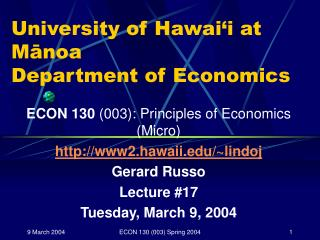Download PresentationUniversity of Hawai‘i at Mānoa Department of Economics

# University of Hawai‘i at Mānoa Department of Economics - PowerPoint PPT PresentationDownload Presentation## University of Hawai‘i at Mānoa Department of Economics

- - - - - - - - - - - - - - - - - - - - - - - - - - - E N D - - - - - - - - - - - - - - - - - - - - - - - - - - -
##### Presentation Transcript

1. University of Hawai‘i at MānoaDepartment of Economics ECON 130 (003): Principles of Economics (Micro) http://www2.hawaii.edu/~lindoj Gerard Russo Lecture #17 Tuesday, March 9, 2004 ECON 130 (003) Spring 2004

2. ANNOUNCEMENTS • REVIEW SESSION • Thursday, March 18, 2004, 4:30-5:30 PM BIL 152 • MID-TERM EXAMINATION #2 • Tuesday, March 30, 2004, 12:00-1:15 PM BIL 152 ECON 130 (003) Spring 2004

3. Cost Curves: Homework ECON 130 (003) Spring 2004

4. Total Cost = 10*Q • Total Fixed Cost = • Total Variable Cost = • Average Total Cost = • Average Fixed Cost = • Average Variable Cost = • Marginal Cost = ECON 130 (003) Spring 2004

5. Total Cost = 10*Q • Total Fixed Cost = 0 • Total Variable Cost = 10*Q • Average Total Cost = 10 • Average Fixed Cost = 0 • Average Variable Cost = 10 • Marginal Cost = 10 ECON 130 (003) Spring 2004

6. Total Cost = 100 + 10*Q • Total Fixed Cost = • Total Variable Cost = • Average Total Cost = • Average Fixed Cost = • Average Variable Cost = • Marginal Cost = ECON 130 (003) Spring 2004

7. Total Cost = 100 + 10*Q • Total Fixed Cost = 100 • Total Variable Cost = 10*Q • Average Total Cost =100/Q + 10 • Average Fixed Cost =100/Q • Average Variable Cost =10 • Marginal Cost =10 ECON 130 (003) Spring 2004

8. Total Cost = 100 + 10*Q + Q2 • Total Fixed Cost = • Total Variable Cost = • Average Total Cost = • Average Fixed Cost = • Average Variable Cost = • Marginal Cost = ECON 130 (003) Spring 2004

9. Total Cost = 100 + 10*Q + Q2 • Total Fixed Cost = 100 • Total Variable Cost = 10*Q + Q2 • Average Total Cost = 100/Q + 10 + Q • Average Fixed Cost = 100/Q • Average Variable Cost = 10 + Q • Marginal Cost = 10 + 2*Q ECON 130 (003) Spring 2004

10. Total Cost = 60*Q – 12*Q2 + Q3 • Total Fixed Cost = • Total Variable Cost = • Average Total Cost = • Average Fixed Cost = • Average Variable Cost = • Marginal Cost = ECON 130 (003) Spring 2004

11. Total Cost = 60*Q – 12*Q2 + Q3 • Total Fixed Cost = 0 • Total Variable Cost = 60*Q – 12*Q2 + Q3 • Average Total Cost = 60 – 12*Q + Q2 • Average Fixed Cost = 0 • Average Variable Cost = 60 – 12*Q + Q2 • Marginal Cost = 60 – 24*Q + 3*Q2 ECON 130 (003) Spring 2004

12. TC = 75 + 14*Q – 6*Q2 + Q3 • Total Fixed Cost = • Total Variable Cost = • Average Total Cost = • Average Fixed Cost = • Average Variable Cost = • Marginal Cost = ECON 130 (003) Spring 2004

13. TC = 75 + 14*Q – 6*Q2 + Q3 • Total Fixed Cost = 75 • Total Variable Cost = 14*Q – 6*Q2 + Q3 • Average Total Cost = 75/Q + 14 – 6*Q + Q2 • Average Fixed Cost = 75/Q • Average Variable Cost = 14 – 6*Q + Q2 • Marginal Cost = 14 – 12*Q + 3*Q2 ECON 130 (003) Spring 2004

14. TC = 50 + 111*Q – 7*Q2 + (1/3)*Q3 • Total Fixed Cost = • Total Variable Cost = • Average Total Cost = • Average Fixed Cost = • Average Variable Cost = • Marginal Cost = ECON 130 (003) Spring 2004

15. TC = 50 + 111*Q – 7*Q2 + (1/3)*Q3 • Total Fixed Cost = 50 • Total Variable Cost = 111*Q – 7*Q2 + (1/3)*Q3 • Average Total Cost = 50/Q + 111 – 7*Q + (1/3)*Q2 • Average Fixed Cost = 50/Q • Average Variable Cost = 111 – 7*Q + (1/3)*Q2 • Marginal Cost = 111 – 14*Q + Q2 ECON 130 (003) Spring 2004